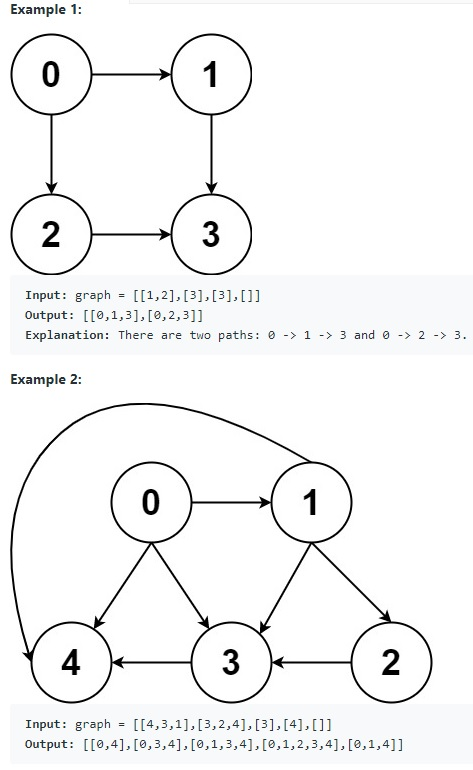# Teaching Kids Programming – Finding All Paths from Source to Target in a Directed Acyclic Graph (DAG) using Recursive Depth First Search Algorithm

Teaching Kids Programming: Videos on Data Structures and Algorithms

Given a directed acyclic graph (DAG) of n nodes labeled from 0 to n – 1, find all possible paths from node 0 to node n – 1 and return them in any order. The graph is given as follows: graph[i] is a list of all nodes you can visit from node i (i.e., there is a directed edge from node i to node graph[i][j]).Example 1:
Input: graph = [[1,2],,,[]]
Output: [[0,1,3],[0,2,3]]
Explanation: There are two paths: 0 -> 1 -> 3 and 0 -> 2 -> 3.

Example 2:
Input: graph = [[4,3,1],[3,2,4],,,[]]
Output: [[0,4],[0,3,4],[0,1,3,4],[0,1,2,3,4],[0,1,4]]

Example 3:
Input: graph = [,[]]
Output: [[0,1]]

Example 4:
Input: graph = [[1,2,3],,,[]]
Output: [[0,1,2,3],[0,2,3],[0,3]]

Example 5:
Input: graph = [[1,3],,,[]]
Output: [[0,1,2,3],[0,3]]

Constraints:
n == graph.length
2 <= n <= 15
0 <= graph[i][j] < n
graph[i][j] != i (i.e., there will be no self-loops).
All the elements of graph[i] are unique.
The input graph is guaranteed to be a DAG.

### Finding All Paths from Source to Target in a Directed Acyclic Graph (DAG) using Recursive Depth First Search Algorithm

Since the Graph is DAG (Directed Acyclic Graph), it does not have cycles. We then can simplify the implementation without needing to use a set to avoid the nodes that we have visisted. We can perform a Depth First Search Algorithm and keep tracking a current path which will be added to the result once we reach the destination.

 ```1 2 3 4 5 6 7 8 9 10 11 12 13 ``` ```class Solution:     def allPathsSourceTarget(self, graph: List[List[int]]) -> List[List[int]]:         ans = []         def dfs(curPath, n):             if n == len(graph) - 1:                 ans.append(curPath[:])                 return                         for nextNode in graph[n]:                 curPath.append(nextNode)                 dfs(curPath, nextNode)                 curPath.pop()                 dfs(, 0)         return ans```
```class Solution:
def allPathsSourceTarget(self, graph: List[List[int]]) -> List[List[int]]:
ans = []
def dfs(curPath, n):
if n == len(graph) - 1:
ans.append(curPath[:])
return
for nextNode in graph[n]:
curPath.append(nextNode)
dfs(curPath, nextNode)
curPath.pop()
dfs(, 0)
return ans```

The space complexity is also O(N) – if we ignore the space required to store all paths (which is N*2^N) as we are using Recursion and need to have a current path list. The time complexity in worst case is O(N*2^N) if each node can be connected to the nodes that have greater values. For example, 0 to 1..n, 1 to 2..n, 3 to 4..n.

Another Python implementation using the Fancy yield (iterator) to implement the Depth First Search Algorithm:

 ```1 2 3 4 5 6 7 8 9 ``` ```class Solution:     def allPathsSourceTarget(self, G: List[List[int]]) -> List[List[int]]:         def dfs(node: int, path: List[int]):             if node == len(G) - 1:                 yield path             else:                 for i in G[node]:                     yield from dfs(i, path + [i])         return [i for i in dfs(0, )]```
```class Solution:
def allPathsSourceTarget(self, G: List[List[int]]) -> List[List[int]]:
def dfs(node: int, path: List[int]):
if node == len(G) - 1:
yield path
else:
for i in G[node]:
yield from dfs(i, path + [i])
return [i for i in dfs(0, )]```

We can also use the Breadth First Search Algorithm to traverse the Graph: Teaching Kids Programming – Finding All Paths from Source to Target in a Directed Acyclic Graph (DAG) using Breadth First Search Algorithm

GD Star Rating